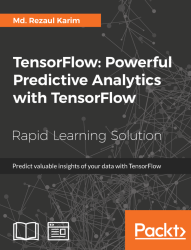•#### TensorFlow: Powerful Predictive Analytics with TensorFlow#### Overview of this book

Predictive analytics discovers hidden patterns from structured and unstructured data for automated decision making in business intelligence. Predictive decisions are becoming a huge trend worldwide, catering to wide industry sectors by predicting which decisions are more likely to give maximum results. TensorFlow, Google’s brainchild, is immensely popular and extensively used for predictive analysis. This book is a quick learning guide on all the three types of machine learning, that is, supervised, unsupervised, and reinforcement learning with TensorFlow. This book will teach you predictive analytics for high-dimensional and sequence data. In particular, you will learn the linear regression model for regression analysis. You will also learn how to use regression for predicting continuous values. You will learn supervised learning algorithms for predictive analytics. You will explore unsupervised learning and clustering using K-meansYou will then learn how to predict neighborhoods using K-means, and then, see another example of clustering audio clips based on their audio features. This book is ideal for developers, data analysts, machine learning practitioners, and deep learning enthusiasts who want to build powerful, robust, and accurate predictive models with the power of TensorFlow. This book is embedded with useful assessments that will help you revise the concepts you have learned in this book. This book is repurposed for this specific learning experience from material from Packt's Predictive Analytics with TensorFlow by Md. Rezaul Karim.
TensorFlow: Powerful Predictive Analytics with TensorFlowCreditsPrefaceFree Chapter
From Data to Decisions – Getting Started with TensorFlowPutting Data in Place – Supervised Learning for Predictive AnalyticsClustering Your Data – Unsupervised Learning for Predictive AnalyticsUsing Reinforcement Learning for Predictive Analytics## Assessments

1. Each tensor is described by a unit of dimensionality called ____.

1. Data type

2. Rank

3. Variables

4. Fetches

2. State whether the following statement is True or False: TensorFlow uses the computation graph to execute an application, where each node represents an operation and the arcs are the data between operations.

3. State whether the following statement is True or False: NumPy has the n–dimensional array support, but it doesn't offer methods to create tensor functions and automatically compute derivatives (+ no GPU support).

4. Which objects does a TensorFlow graph contains?

5. When you're performing a little complex operation with TensorFlow, for example training a linear regression, TensorFlow internally represents its computation using a dataﬂow graph. The graph is called as?

1. Dataflow graph

2. Linear graph

3. Computational graph

4. Regression graph